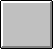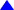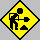Learning Statistics with SPSS / HYPOTHESIS TESTING

# Hypothesis Testing

Contents:                      One-Sample T-Test
Two-Samples T-Test: Independent Samples
Paired-Samples T-Test: Dependent Samples
Chi-Square Test of Independence
One-Way ANOVA or F-Test
Non-Parametric Tests
Other Tests

###See ExampleONE-SAMPLE T-TEST

[Command Sequence: Analyze/Compare Means/One-Sample T Test/Options/                                                Confidence Interval]

Note: Select variable and test value at the One-Sample T test window. You may optionally supply your own level of significance (the default value is .05 because the default confidence level is .95) in the Confidence Interval box after opening the Options window.Top

###See ExampleTWO-SAMPLES T-TEST: INDEPENDENT SAMPLES

[Command Sequence: Analyze/Compare Means/Independent-Samples T Test/                                                Define Groups/Options/Confidence Interval]

Note: At the Independent-Samples T Test move the test variable into the Test Variable(s) box and the grouping variable into the Grouping Variable. Click on Define Group button and specify the value for group 1 (e.g., yes) and the value for group 2 (e.g., no). Then click on the Options button and set the level of significance (the default confidence level of 95% implies that the level of significance is 5%).Top

###See ExamplePAIRED-SAMPLES T-TEST: DEPENDENT SAMPLES

[Command Sequence: Analyze/Compare Means/Paired-Samples T Test/                                                 Paired Variables/Options/Confidence Interval]

Note
: At the Paired-Samples T Test window fill the Current Selections box by clicking on the pseudo variables (e.g., Before and After) one at a time. The first (e.g., After) is labeled as Variable 1, and the second (e.g., Before) is labeled as Variable 2. Click on the arrow (now pointing to the right) to define the difference di = After - Before in the Paired Variable box. Open the Options menu and set the level of significance (the default confidence level of 95% implies that the level of significance is 5%).Top

###See ExampleCHI-SQUARE TEST OF INDEPENDENCE

[Command Sequence: Analyze/Summarize/Crosstabs]

Note: At the Crosstabs windows 1) move one variable Rows box and the other to the Columns box; 2) Open Statistics window and check the Chi-Square box; 3) open the Cells window and check the Expected box; 4) optionally you may check Rows, Columns, Totals boxes under Percentages.

###See ExampleONE-WAY ANOVA OR F-TEST

[Command Sequence: Analyze/Compare Means/One-Way ANOVA]

Note: At the One-Way window do the following: (1) move the Response Variable (Dependent Variable) to the Dependent List box, (2) move the Factor (Independent Variable) to the Factor box, (3) open the Options window and check the Statistics box.

###See ExampleNON-PARAMETRIC TESTS

[Command Sequence: Statistics/...]Top

###See ExampleOTHER TESTS

[Command Sequence: Analyze/...]

Note:Top or Back to Learning Statistics with SPSS/win or Home page or Send me your Comments.